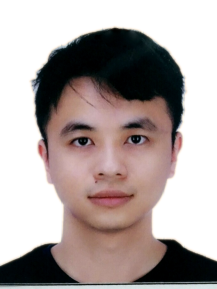# Chuangqiang HuChuangqiang Hu (胡创强)

Assistant Research Fellow

Email: huchq@bimsa.cn

Research Fileld: Coding theory and Singularity theory

In coding theory, we constructed multi-point AG codes over BBGS tower, GS tower, Weierstrass extension fields, GGS curves, etc. Our basis technique is to describe the Riemann-Roch space by means of lattice point set. With the good basis constructed, we are able to calculate the Weierstrass pure gaps explicitly.

In singularity theory, we introduce the k-th Tjurina algebras and the k-th Yau algebras derived from isolated hypersurface singularities. It establishes the natural relation between {isolated hypersurface singularities of fixed dimension} and {finite dimensional solvable/nilpotent Lie algebras}. Along this line, we proved some Torelli-type theorems for simple elliptic singularities ~E_6, ~E_7 and ~E_8.

In number Theory, we obtain equations of rank-m Drinfeld modular curves, with m >= 2. The resulting equations coincide with the BBGS towers.